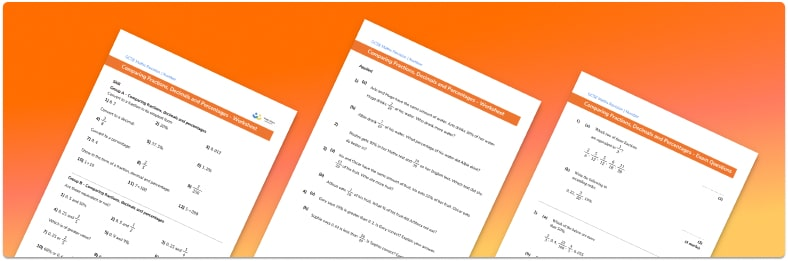# Comparing Fractions Decimals And Percentages Worksheet• Section 1 of the comparing fractions, decimals and percentages worksheet contains 36 skills based comparing fractions, decimals and percentages questions, in 3 groups to support differentiation
• Section 2 contains 4 applied comparing fractions, decimals and percentages questions with a mix of worded problems and deeper problem solving questions
• Section 3 contains 4 foundation and higher level GCSE exam style comparing fractions, decimals and percentages questions
• Answers and a mark scheme for all comparing fractions, decimals and percentages questions
• Follows variation theory with plenty of opportunities for students to work independently at their own level
• All questions created by fully qualified expert secondary maths teachers
• Suitable for GCSE maths revision for AQA, OCR and Edexcel exam boards

• This field is for validation purposes and should be left unchanged.

You can unsubscribe at any time (each email we send will contain an easy way to unsubscribe). To find out more about how we use your data, see our privacy policy.

### Comparing fractions, decimals, and percentages at a glance

Fractions, decimals and percentages (FDP) are all different formats for representing a part of a whole. We sometimes want to convert between different data formats to make data easier to compare or work with.

Ordering fractions, decimals & percentages can be carried out by converting each number to the same form and comparing place value. Once all of the numbers are in the same form, ordering decimals and ordering percentages can be carried out by simply ordering the numbers. Ordering fractions can be more difficult as they may need to be written in their simplest form or as an equivalent fraction to ensure they all have the same denominator.

Converting from percentages to decimals is carried out by dividing by 100 and conversely, converting from decimals to percentages is carried out by multiplying by 100.

Converting between fractions and decimals is carried out by dividing the numerator and denominator of the fraction to give a decimal. Conversely we can convert a decimal to  fraction by multiplying the decimal number by 100 to first give a percentage and then writing the percentage as a fraction over 100.

Converting fractions to percentages can be done by using equivalent fractions to find a fraction with a denominator of 100; the numerator is the percentage. Alternatively we can first convert fractions to decimals by dividing the numerator by the denominator and then multiply the result by 100 to give a percentage.

Once all of the data is in the same form we can compare or order it.

Looking forward, students can then progress to additional number worksheets, for example aor aFor more teaching and learning support on Number our GCSE maths lessons provide step by step support for all GCSE maths concepts.

## Do you have KS4 students who need more focused attention to succeed at GCSE?There will be students in your class who require individual attention to help them succeed in their maths GCSEs. In a class of 30, it’s not always easy to provide.

Help your students feel confident with exam-style questions and the strategies they’ll need to answer them correctly with our dedicated GCSE maths revision programme.

Lessons are selected to provide support where each student needs it most, and specially-trained GCSE maths tutors adapt the pitch and pace of each lesson. This ensures a personalised revision programme that raises grades and boosts confidence.

Find out more